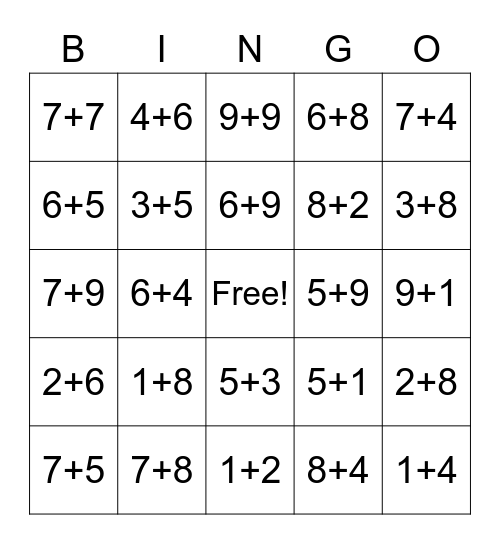# Math BingoThis bingo card has a free space and 24 words: 7+5, 1+8, 6+9, 8+4, 7+4, 7+7, 4+6, 9+9, 5+9, 9+1, 7+9, 3+5, 5+1, 2+8, 6+5, 7+8, 1+2, 6+8, 3+8, 2+6, 6+4, 5+3, 8+2 and 1+4.

## Play Online